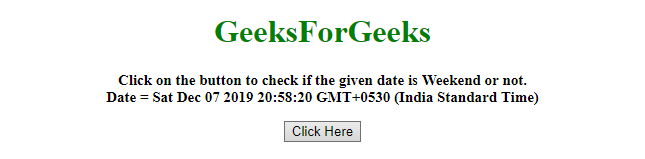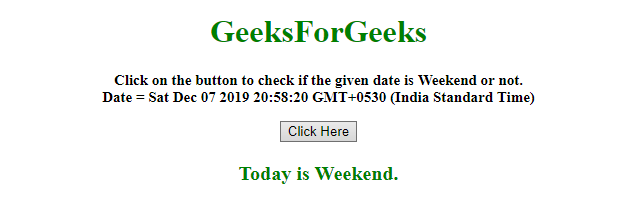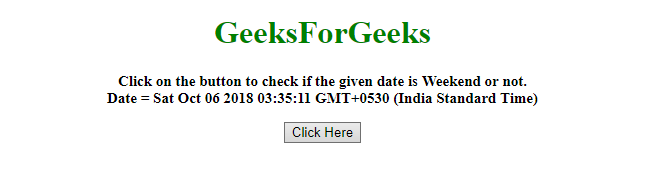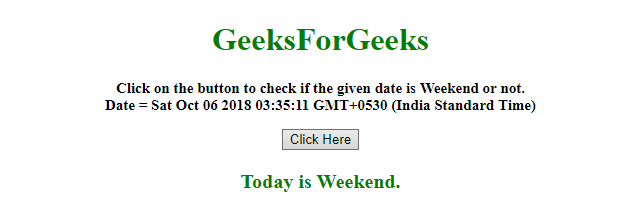Skip to content
Related Articles
How to check if the given date is weekend ?
• Last Updated : 13 Dec, 2019

Given a date and the task is to determine if the given date is weekend (In this case we are considering Saturday as weekend). There are two methods to solve this problem which are discussed below:

Approach 1:

• Use .getDay() method on the Date object to get the day.
• Check if it is 6 or not (6 denotes Saturday).

Example: This example implements the above approach.

 ` ``<``html``> `` ` `<``head``> ``    ``<``title``> ``        ``How to check if the given``        ``date is weekend ?     ``    ```` `` ` `<``body` `style` `= ``"text-align:center;"``> ``     ` `    ``<``h1` `style` `= ``"color:green;"` `> ``        ``GeeksForGeeks ``    ````     ` `    ``<``p` `id` `= ``"GFG_UP"` `style` `=``        ``"font-size: 15px; font-weight: bold;"``>``    ````     ` `    ``<``button` `onclick` `= ``"gfg_Run()"``> ``        ``Click Here``    ````     ` `    ``<``p` `id` `= ``"GFG_DOWN"` `style = "color:green;``        ``font-size: 20px; font-weight: bold;">``    ````     ` `    ``<``script``>``        ``var el_up = document.getElementById("GFG_UP");``        ``var el_down = document.getElementById("GFG_DOWN");``        ``var date = new Date();``         ` `        ``el_up.innerHTML = "Click on the button to "``                ``+ "check if the given date is Weekend"``                ``+ " or not.<``br``>Date = " + date;``         ` `        ``function gfg_Run() {``            ``var day = date.getDay();``            ``var ans = (day === 6);``             ` `            ``if (ans) {``                ``ans = "Today is Weekend.";``            ``} else {``                ``ans = "Today is not Weekend.";``            ``}``            ``el_down.innerHTML = ans;``        ``} ``    `` `` `` ` ``

Output:

• Before clicking on the button:• After clicking on the button:Approach 2:

• Use .toString() method to convert the Date object to string.
• Get the String from the date object using .substring() method.
• Compare it to the string “Sat” to get the answer.

Example: This example implements the above approach.

 ` ``<``html``> `` ` `<``head``> ``    ``<``title``> ``        ``How to check if the given``        ``date is weekend ?``    ```` `` ` `<``body` `style` `= ``"text-align:center;"``> ``     ` `    ``<``h1` `style` `= ``"color:green;"` `> ``        ``GeeksForGeeks ``    ````     ` `    ``<``p` `id` `= ``"GFG_UP"` `style` `= ``        ``"font-size: 15px; font-weight: bold;"``>``    ````     ` `    ``<``button` `onclick` `= ``"gfg_Run()"``> ``        ``Click Here``    ````     ` `    ``<``p` `id` `= ``"GFG_DOWN"` `style = "color:green;``        ``font-size: 20px; font-weight: bold;">``    ````     ` `    ``<``script``>``        ``var el_up = document.getElementById("GFG_UP");``        ``var el_down = document.getElementById("GFG_DOWN");``        ``var date = new Date(1538777111111);``         ` `        ``el_up.innerHTML = "Click on the button to "``                ``+ "check if the given date is Weekend"``                ``+ " or not.<``br``>Date = " + date;``         ` `        ``function gfg_Run() {``            ``var day = date.toString();``             ` `            ``if (day.substring(0, 3) === "Sat") {``                ``ans = "Today is Weekend.";``            ``} else {``                ``ans = "Today is not Weekend.";``            ``}``            ``el_down.innerHTML = ans;``        ``} ``    `` `` `` ` ``

Output:

• Before clicking on the button:• After clicking on the button:My Personal Notes arrow_drop_up